﻿ Physical Property Estimation: Online Demonstration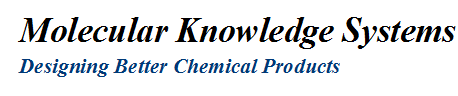Physical Property Estimation

There are numerous physical property estimation techniques which accurately predict a chemical's or mixture's physical properties. These techniques can be classified into two broad categories: group contribution techniques and equation oriented techniques. Group contribution estimation techniques begin with a compound's molecular structure. A "contribution" for each group found in the structure is totaled into an estimate. Once several basic properties are estimated by group contribution techniques these can be entered into equation oriented techniques for further property estimation.

This webpage provides descriptions and calculation capabilities for a number of commonly used physical properties. For additional physical property estimation capabilities please download our Cranium Demo. Cranium goes far beyond this online estimation demonstration enabling you to draw molecular structures, enter your own estimation techniques, estimate mixture properties and create your own databases.

We are always happy to discuss physical properties. Please your comments and suggestions.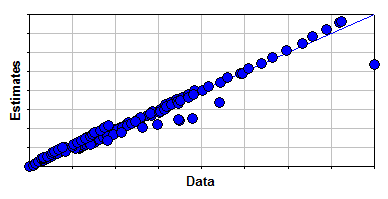Figure 1: Estimation Errors for High Pressure Vapor Thermal Conductivity Data
Structure Entry

Currently our web-based estimation requires you to manually enter the number of groups which occur within the structure being estimated. For example, the molecular structure of 1-butanol is shown in Figure 1. To estimate properties for 1-butanol you would:

• choose a "-CH3" group and enter a 1 for the occurrence
• choose a "-CH2-" group and enter a 3 for the occurrence
• choose a "-OH (alcohol)" and enter a 1 for the occurrence.

This group dissection is shown in the Figure 2. Use the controls below to show the group dissections of several other common chemicals:

 1-Butanol Acetone Methanol n-Hexane

Once you have entered your structure's groups, press the "Estimate Properties" button below and scroll the window to examine estimated property values. Press the "Clear Occurrences" button to set all group occurrences to zero.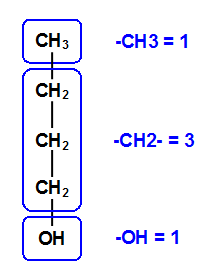Figure 2: Group Dissection of
1-Butanol
Basic Properties Estimates

Some of the most basic properties needing to be estimated for pure chemicals are the molecular weight, the melting point and the normal boiling point. The basic estimation models for these properties are shown in Figure 3.

• The molecular weight is estimated by its definition - the molecular weight is the sum of the atomic weight of each atom in the molecule.
• The melting point is estimated by Joback's method , a group contribution technique. Melting point estimates are not highly accurate - the average absolute percent error is 15.8%.
• The normal boiling point is estimated by Joback's method , a group contribution technique. Boiling point estimates have an average absolute percent error of 5.3%.

Click on the report links in the following table for more detailed evaluations of the estimation techniques.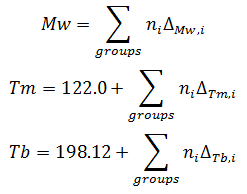Figure 3: Basic Properties Models
 Property Technique Estimated Value Units Report Molecular Weight Definition - - - - - - Melting Point Joback Method K Tm Report Boiling Point Joback Method K Tb Report
Critical Properties Estimates

The critical properties are used to define the chemical's liquid and vapor region. They are also used in many other estimation techniques. The estimation models for the critical properties are shown in Figure 4.

• The critical temperature is estimation by Joback's method , a group contribution technique. The boiling point is also a required input. Critical temperature estimates have an average absolute percent error of 2.2%.
• The critical pressure is estimated by Lydersen's method , a group contribution technique. The molecular weight is also a required input. Critical pressure estimates have an average absolute percent error of 6.9%.
• The critical volume is estimated by Joback's method , a group contribution technique. Critical volume estimates have an average absolute percent error of 3.6%.
• The critical compressibility is estimated using its definition. The critical temperature, critical pressure and critical volume are required inputs.

Click on the report links in the following table for more detailed evaluations of the estimation techniques.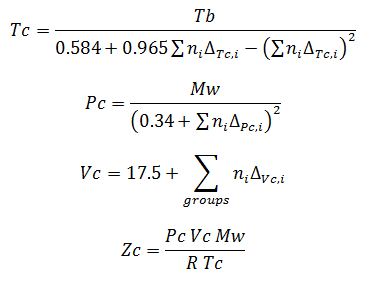Figure 4: Critical Properties Models
 Property Technique Estimated Value Units Report Critical Temperature Joback Method K Tc Report Critical Pressure Lydersen Method Pa Pc Report Critical Volume Joback Method m3/kg Vc Report Critical Compressibility Definition - - - - - -
 Liquid Density Estimates The liquid density is estimated at several temperatures between the normal boiling point and the critical temperature by the Rackett equation . This technique is an equation oriented technique that requires the molecular weight, the critical compressibility, the critical temperature and the critical pressure. These properties are obtained from previously estimated values. Estimates generated by the Racket equation have an average absolute percent error of 5.7%. See this report for a more detailed evaluation of the technique.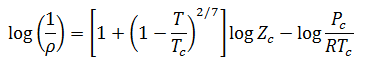Figure 5: Rackett Equation
 Temperature [K] Estimate [kg/m3]
 Temperature [K] Estimate [kg/m3]
 Vapor Pressure Estimates The vapor pressure is estimated at several temperatures between the normal boiling point and the critical temperature by the Lee-Kesler equation . This technique is an equation oriented technique that requires the critical temperature, critical pressure and acentric factor. The acentric factor is found using these same equations with the temperature equal to the boiling point and the vapor pressure equal to 1 atm. The remaining properties are obtained from previously estimated values. Estimates generated by the Lee-Kesler equation have an average absolute percent error of 12.8%. This technique does not estimate low vapor pressure values well. The error estimate was calculated for estimates greater than 10,000 Pa. See this report for a more detailed evaluation of the technique.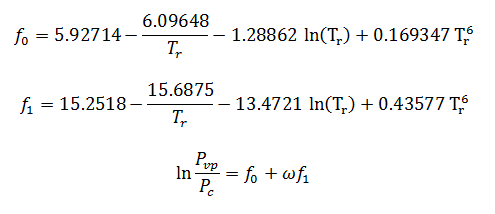Figure 6: Lee-Kesler Equation
 Temperature [K] Estimate [Pa]
 Temperature [K] Estimate [Pa]
References
 1) Kevin G. Joback and Robert C. Reid. "Estimation of Pure-Component Properties from Group-Contributions." Chemical Engineering Communications. Volume 57, page 233-243, 1987. 2) Harold G. Rackett. "Equation of State for Saturated Liquids." Journal of Chemical and Engineering Data. Volume 15, number 4, page 514 - 517, 1970. 3) A. L. Lydersen. "Estimation of Critical Properties of Organic Compounds." Engineering Experiment Station Report 3. April 1955. 4) Byung I. Lee and Michael G. Kesler. "A Generalized Thermodynamic Correlation based on Three-Parameter Corresponding States." AIChE Journal. Volume 21, number 3, page 510-527, 1975.